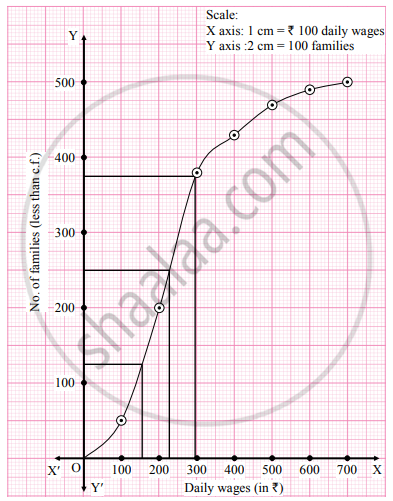# The following table gives the distribution of daily wages of 500 families in a certain city. Daily wages No. of families Below 100 50 100 – 200 150 200 – 300 180 300 – 400 50 400 – 500 40 500 - Mathematics and Statistics

Graph

The following table gives the distribution of daily wages of 500 families in a certain city.

 Daily wages No. of families Below 100 50 100 – 200 150 200 – 300 180 300 – 400 50 400 – 500 40 500 – 600 20 600 above 10

Draw a ‘less than’ ogive for the above data. Determine the median income and obtain the limits of income of central 50% of the families.

#### Solution

To draw a ogive curve, we construct the less than cumulative frequency table as given below:

 Daily wages No. of families (f) Less than cumulative frequency(c.f.) Below 100 50 50 100 – 200 150 200 200 – 300 180 380 300 – 400 50 430 400 – 500 40 470 500 – 600 20 490 600 above 10 500 Total 500

The points to be plotted for less than ogive are (100, 50), (200, 200), (300, 380), (400, 430), (500, 470), (600, 490) and (700, 500).Here, N = 500 = 125

For Q1, "N"/4=500/4 = 250

For Q2, "N"/2=500/2

For Q3, "3N"/4=(3xx500)/4 = 375

∴ We take the points having Y coordinates 125, 250, and 375 on Y-axis. From these points, we draw lines parallel to X-axis. From the points where these lines intersect the curve, we draw perpendiculars on X-axis. X-Co-ordinates of these points gives the values of Q1, Q2, and Q3.

∴ Q1 ≈ 150, Q2 ≈ 228, Q3 ≈ 297

∴ Median = 228
50% of families lies between Q1 and Q3
∴ Limits of income of central 50% of families are from ₹ 150 to ₹ 297.

Concept: Graphical Location of Partition Values
Is there an error in this question or solution?